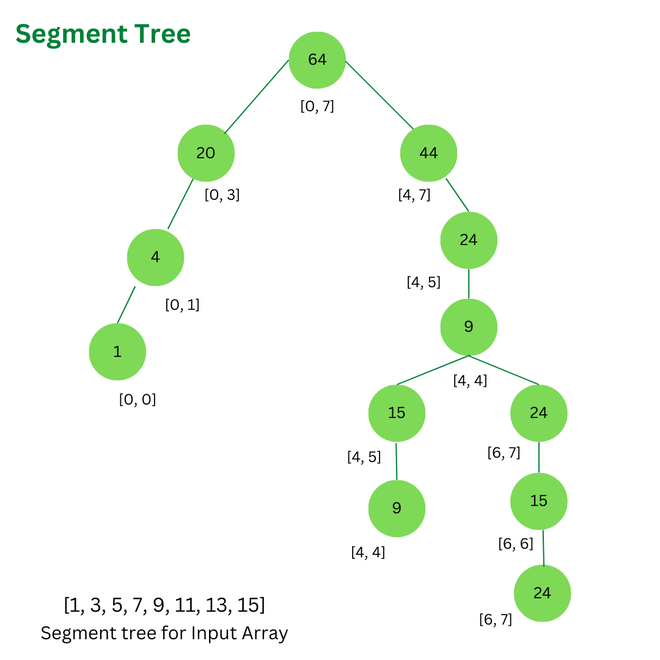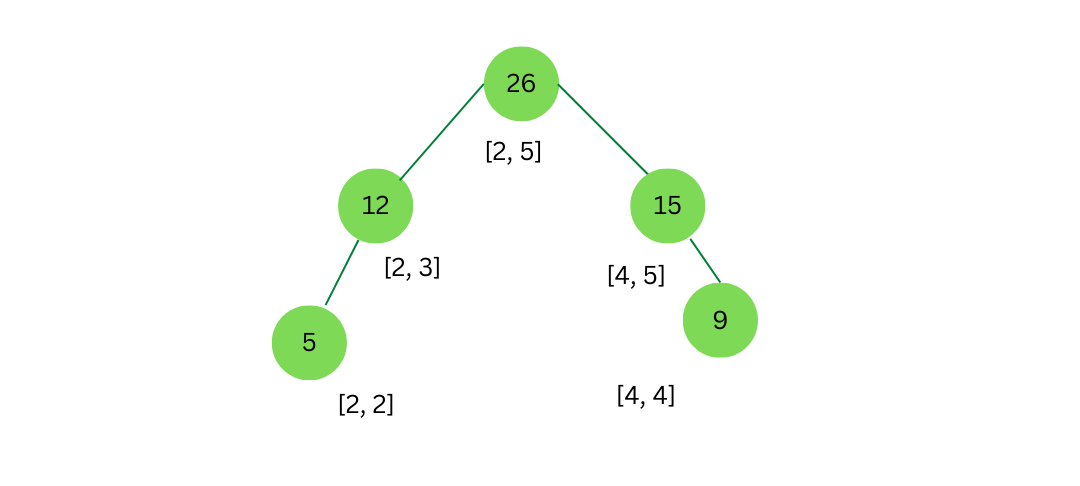GeeksforGeeks App
Open AppBrowser
Continue

# Generalizing Segment Trees with Rust

### Segment Tree

A segment tree is a data structure that can be used to efficiently store and query information about ranges in an array. It is a balanced binary tree, where each node represents a range of the array and stores some aggregated information about that range. Segment trees can be used to support various operations, such as finding the minimum or maximum element in a range, summing up the elements in a range, or checking whether all elements in a range satisfy a certain condition.

In Rust, segment trees can be implemented using a generic approach, which allows them to be used with any type of element and any type of aggregated information. Here is a general outline of how to implement a segment tree in Rust:

• Define a struct for the segment tree nodes. The struct should have fields for the range of the array represented by the node, the aggregated information about the range, and pointers to the left and right children of the node.
• Implement a function for creating a new segment tree node. This function should take as input the range of the array represented by the node and the aggregated information about the range, and should return a new segment tree node with the appropriate fields initialized.
• Implement a function for building the segment tree. This function should take as input the array and a range representing the portion of the array that the tree should cover. It should recursively build the tree by dividing the range into smaller ranges and creating new nodes for each range.
• Implement functions for querying the segment tree. These functions should take as input a query range and should return the aggregated information about that range by traversing the tree and combining the information stored in the nodes that overlap with the query range.
• (Optional) Implement functions for updating the segment tree. These functions should allow the user to modify the elements of the array and should update the aggregated information in the tree accordingly.

Here is an example of how a segment tree might be structured to represent an array of 8 elements:In this diagram, each node represents a range of the array and stores some aggregated information about that range. The root node represents the entire array, and the child nodes represent subranges of the array.

The values at each node represent the sum of the elements in the range represented by the node.
For example, the root node represents the range [0, 7] and has a value of 64, which is the sum of all the elements in the array [1, 3, 5, 7, 9, 11, 13, 15]. The left child of the root node represents the range [0, 3] and has a value of 20, which is the sum of the elements in the range [1, 3, 5, 7]. The right child of the root node represents the range [4, 7] and has a value of 44, which is the sum of the elements in the range [9, 11, 13, 15].

To query the segment tree for information about a specific range, we can use a process similar to the one shown in the following diagram:In this tree, each node represents a range of the array and the sum field of the node represents the sum of the elements in the range.

For example, the root node represents the range [0, 7] and has a sum field with a value of 64, which is the sum of all the elements in the array [1, 3, 5, 7, 9, 11, 13, 15]. The left child of the root node represents the range [0, 3] and has a sum field with a value of 20, which is the sum of the elements in the range [1, 3, 5, 7]. The right child of the root node represents the range [4, 7] and has a sum field with a value of 44, which is the sum of the elements in the range [9, 11, 13, 15].The second tree in the diagram represents a subrange of the first tree, specifically the range [2, 5]. The root node of this tree represents the range [2, 5] and has a sum field with a value of 26, which is the sum of the elements in the range [5, 7, 9, 11]. The left child of the root node represents the range [2, 3] and has a sum field with a value of 12, which is the sum of the elements in the range [5, 7]. The right child of the root node represents the range [4, 5] and has a sum field with a value of 15, which is the sum of the elements in the range [9, 11].

### Implementation:

This implementation defines a `Node` struct for the segment tree nodes, with fields for the range of the array represented by the node, the sum of the elements in the range, and pointers to the left and right children of the node. The `Node` struct also has three methods:

– `new`: creates a new node with the given range and sum
– `build`: builds the segment tree by recursively dividing the array into smaller ranges and creating new nodes for each range
`query`: queries the segment tree for the sum of elements in a given range by traversing the tree and combining the information stored in the overlapping nodes

• The `build` method takes as input the array and a range representing the portion of the array that the tree should cover, and it returns an `Option<Box<Node<T>>>` representing the root node of the tree.
• If the range is empty, it returns `None`. Otherwise, it creates a new node for the range and recursively calls itself on the left and right halves of the range to build the left and right children of the node.
• The `query` method takes as input a query range and returns the sum of elements in the range by traversing the tree and combining the information stored in the overlapping nodes.

Here is an example of how a segment tree might be implemented in Rust to support querying the sum of elements in a range:

## Rust

 `use` `std::cmp::{max, min};` `struct` `Node {``  ` `    ``// Range of the array represented by the node``    ``range: (usize, usize),``  ` `    ``// Sum of the elements in the range``    ``sum: T,``  ` `    ``// Left child node``    ``left: Option>>,``  ` `    ``// Right child node``    ``right: Option>>,``}` `impl``> Node {``    ` `    ``// Function to create a new node``    ``fn` `new(range: (usize, usize), sum: T) -> ``Self` `{``        ``Self` `{ range, sum, left: None, right: None }``    ``}` `    ``// Function to build the segment tree``    ``fn` `build(arr: &[T], range: (usize, usize)) -> Option> {``        ``if` `range.0 > range.1 {``            ``return` `None;``        ``}``        ``let` `mid = (range.0 + range.1) / 2;``        ``let` `mut` `node = ``Self``::new(range, T::clone(&arr[range.0]));``        ``if` `range.0 < range.1 {``            ``node.left = ``Self``::build(arr, (range.0, mid));``            ``node.right = ``Self``::build(arr, (mid + 1, range.1));``            ``node.sum = node.sum + node.left.as_ref().map(|x| x.sum.clone()).unwrap_or(T::clone(&arr[range.0]));``            ``node.sum = node.sum + node.right.as_ref().map(|x| x.sum.clone()).unwrap_or(T::clone(&arr[range.0]));``        ``}``        ``Some(Box::new(node))``    ``}` `    ``// Function to query the segment tree``    ``fn` `query(&``self``, range: (usize, usize)) -> T {``        ``if` `range.0 <= ``self``.range.0 && ``self``.range.1 <= range.1 {``            ``return` `self``.sum.clone();``        ``}``        ``let` `mut` `sum = T::clone(&``self``.sum);``        ``if` `let` `Some(``ref` `left) = ``self``.left {``            ``if` `range.0 <= left.range.1 {``                ``sum = sum + left.query((max(range.0, left.range.0), min(range.1, left.range.1)));``            ``}``        ``}``        ``if` `let` `Some(``ref` `right) = ``self``.right {``            ``if` `range.0 <= right.range.1 {``                ``sum = sum + right.query((max(range.0, right.range.0), min(range.1, right.range.1)));``            ``}``        ``}``        ``sum``    ``}``}` `fn` `main() {``    ``let` `arr = [1, 3, 5, 7, 9, 11, 13, 15];``    ``let` `tree = Node::build(&arr, (0, arr.len() - 1));` `    ``// Query the sum of elements in the range [2, 5]``    ``let` `sum = tree.as_ref().unwrap().query((2, 5));``    ``println!(``"The sum of elements in the range [2, 5] is: {}"``, sum);``}`

To use this implementation, we can call the `build` method to build the segment tree and then call the `query` method on the root node to query the tree for the sum of elements in a given range.

For example, the following code queries the segment tree for the sum of elements in the range [2, 5]:

## Rust

 `let` `arr = [1, 3, 5, 7, 9, 11, 13, 15];``let` `tree = Node::build(&arr, (0, arr.len() - 1));` `// Query the sum of elements in the range [2, 5]``let` `sum = tree.as_ref().unwrap().query((2, 5));``println!(``"The sum of elements in the range [2, 5] is: {}"``, sum);`

This implementation can be generalized to work with any type of element and any type of aggregated information by replacing the `T` type parameter with the appropriate type and implementing the necessary trait bounds.

For example, to implement a segment tree that stores the minimum element in a range, we could use the following definition for the `Node` struct:

## Rust

 `impl``> Node {``    ``// function to build the segment tree``    ``fn` `build(arr: &[T], range: (usize, usize)) -> Option> {``        ``if` `range.0 > range.1 {``            ``return` `None;``        ``}``        ``let` `mid = (range.0 + range.1) / 2;``        ``let` `mut` `node = ``Self``::new(range, T::clone(&arr[range.0]));``        ``if` `range.0 < range.1 {``            ``node.left = ``Self``::build(arr, (range.0, mid));``            ``node.right = ``Self``::build(arr, (mid + 1, range.1));``            ``node.sum = node.sum + node.left.as_ref().map(|x| x.sum.clone()).unwrap_or(T::clone(&arr[range.0]));``            ``node.sum = node.sum + node.right.as_ref().map(|x| x.sum.clone()).unwrap_or(T::clone(&arr[range.0]));``        ``}``        ``Some(Box::new(node))``    ``}` `    ``// function to query the segment tree``    ``fn` `query(&``self``, range: (usize, usize)) -> T {``    ``if` `range.0 <= ``self``.range.0 && ``self``.range.1 <= range.1 {``        ``return` `self``.sum.clone();``    ``}``    ``let` `mut` `sum = T::clone(&``self``.sum);``    ``if` `let` `Some(``ref` `left) = ``self``.left {``        ``if` `range.0 <= left.range.1 {``            ``sum = sum + left.query((max(range.0, left.range.0), min(range.1, left.range.1)));``        ``}``    ``}``    ``if` `let` `Some(``ref` `right) = ``self``.right {``        ``if` `range.0 <= right.range.1 {``            ``sum = sum + right.query((max(range.0, right.range.0), min(range.1, right.range.1)));``        ``}``    ``}``    ``sum``}`

### Conclusion:

In conclusion, segment trees are a useful data structure for efficiently answering queries about the ranges of an array. They can be implemented in Rust by defining a struct for the segment tree nodes and implementing the necessary methods for building and querying the tree.

• The Node struct should have fields for the range of the array represented by the node, the aggregated information of interest (e.g., the sum of the elements in the range), and pointers to the left and right children of the node.
• The build method should take as input the array and a range representing the portion of the array that the tree should cover, and it should return an Option<Box<Node<T>>> representing the root node of the tree.
• The query method should take as input a query range and return the aggregated information of interest by traversing the tree and combining the information stored in the overlapping nodes.

This implementation can be generalized to work with any type of element and any type of aggregated information by replacing the T type parameter with the appropriate type and implementing the necessary trait bounds.

Related Articles:

My Personal Notes arrow_drop_up